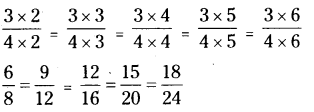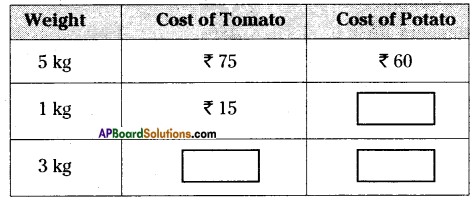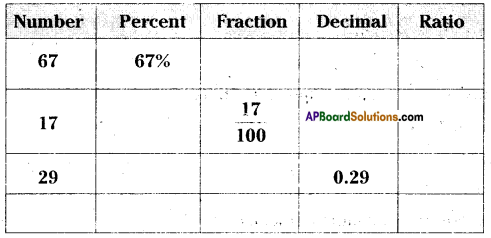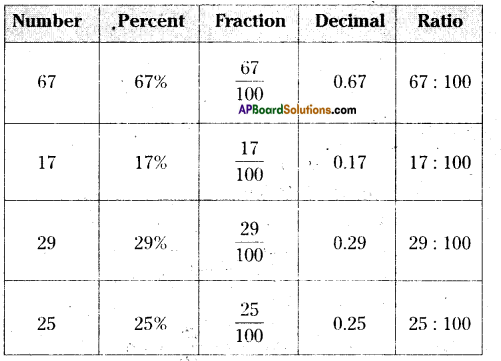AP State Syllabus AP Board 6th Class Maths Solutions Chapter 6 Basic Arithmetic InText Questions and Answers.

## AP State Syllabus 6th Class Maths Solutions 6th Lesson Basic Arithmetic InText QuestionsCheck Your Progress (Page No. 84)

Question 1.
Express the terms 45 and 70 by using ratio symbol.
Solution:
Given terms are 45 and 70
Ratio = 45 : 70
It can be read as 45 is to 70.

Question 2.
Write antecedent in the ratio 7:15.
Solution:
Given ratio 7 : 15
In the ratio first term is called antecedent.
In 7 : 15 antecedent is 7.

Question 3.
Write the consequent in the ratio 8 : 13.
Solution:
Given ratio 8 : 13
In the ratio second term is called consequent.
In 8 : 13 consequent is 13.Question 4.
Express the ratio 35 : 55 in the simplest form.
Solution:
Given ratio 35 : 55 (or)
To write the ratio in the simplest form we have to divide by the common factor of two terms 35 and 55.
Common factor is 5.
Now divide by 5,
$$\frac{35}{55}=\frac{35 \div 5}{55 \div 5}=\frac{7}{11}$$
Simplest form of  is $$\frac{7}{11}$$

Question 5.
In the given figure, find the ratio of
ii) Shaded part to total parts,
iii) Unshaded parts to total parts.Solution:
i) In the given figur.e,
Number of shaded parts = 1
Number of unshaded parts = 3

ii) Number of shaded parts = 1
Total parts = 4
Ratio = shaded parts : total parts = 1:4

iii) Number of unshaded parts = 3
Total parts = 4
Ratio = unshaded parts : total parts = 3:4

Question 6.
Express the following in the form of ratio.
a) The length of a rectangle is triple its breadth. ‘
b) In a school, the workload of teaching 19 sections has been assigned to 38 teachers.
Solution:
a) Let breadth of rectangle = x or one part = 1 part
length of rectangle = triple the breadth
= 3 x x = 3x = 3 parts
Ratio = l : b = x : 3x =$$\frac{1 x}{3 x}=\frac{1}{3}$$ = 1:3

b) Given number of sections = 19
Number of teachers = 38
Ratio = 19 : 38 = $$\frac{19}{38}=\frac{1}{2}$$ = 1 : 2(Page No. 88)

Question 1.
Which ratio is larger in the following pairs ?
(a) 5 : 4 or 9 : 8
(b) 12 : 14 or 16 : 18
(c) 8: 20 or 12: 15
(d)4:7 or 7:11
Solution:
a) 5 : 4 or 9 : 8
Write the given ratios as fractions, we have 5 : 4 = $$\frac{5}{4}$$ and 9 : 8 = $$\frac{9}{8}$$
Now find the LCM of the denominators of 4 and 8 is 8.
Make the denominator of the each fraction equal to 8.
We have $$\frac{5}{4} \times \frac{2}{2}=\frac{10}{8}$$ and $$\frac{9}{8} \times \frac{1}{1}=\frac{9}{8}$$
Clearly we know that 10 > 9
∴ $$\frac{10}{8}>\frac{9}{8}$$ (or) 10 : 8 > 9 : 8
10 : 8 is equal to 5 : 4
Therefore the larger ratio is 5 : 4.

b) 12 : 14 or 16:18
12 : 14 = $$\frac{12}{14}=\frac{6}{7}$$ and 16 : 18 = $$\frac{16}{18}=\frac{8}{9}$$
Now find the LCM of the denominators of 7 and 9 is 63.
Make the denominator of the each fraction equal to 63.
we have $$\frac{6}{7} \times \frac{9}{9}=\frac{54}{63}$$ and $$\frac{8}{9} \times \frac{7}{7}=\frac{56}{63}$$
Clearly, we know that 54 < 56
∴ $$\frac{54}{63}<\frac{56}{63}$$ (or) 54:63 < 56:63
56 : 63 is equal to 16 : 18 (or) 8 : 9
∴ The larger ratio is 16 : 18.

c) 8 : 20 or 12 : 15
Write the given ratios as fractions we have
8:20 = $$\frac{8}{20}=\frac{2}{5}$$ and 12:15 = $$\frac{12}{15}=\frac{4}{5}$$
$$\frac{2}{5}$$ and $$\frac{4}{5}$$

Clearly $$\frac{2}{5}$$ < $$\frac{4}{5}$$
i.e., 2:5 < 4 : 5 (or) 8: 20 < 12: 15
Therefore the larger ratio is 12 : 15.d) 4: 7 or 7: 11
Write the given ratios as fractions, we have 4 7
4 : 7 = $$\frac{4}{7}$$ and 7:11 = $$\frac{7}{11}$$ .
Now find the LCM of the denominators of 7 and 11 is 77.
Make the denominators of the each fraction equal to 77.
We have $$\frac{4}{7} \times \frac{11}{11}=\frac{44}{77}$$ and $$\frac{7}{11} \times \frac{7}{7}=\frac{49}{77}$$
$$\frac{44}{7}$$ and $$\frac{49}{77}$$
Clearly we know that 44 < 49
∴ $$\frac{44}{77}<\frac{49}{77}$$ (or) 44 : 77 < 49 : 77
i.e.,4: 7 < 7 : 11
Therefore the larger ratio is 7 : 11

Question 2.
Find three equivalent ratios of 12 : 16.
Solution:
Given ratio is 12 : 16
Write the given ratio as fraction we have 12:16= $$\frac{12}{16}=\frac{3}{4}$$
Now, write equivalent fractions of $$\frac{3}{4}$$i. e., 6 : 8 = 9 : 12 = 12 : 16 = 15 : 20 = 18 : 24
∴ Equivalent ratios of 12 : 16 are 6 : 8, 9 : 12, 12 : 16, 15 : 20 and 18 : 24.(Page No. 90)

Question 1.
Check whether the following terms are in proportion ?
1) 5,6,7,8
2) 3,5,6,10
3) 4,8,7,14
4) 2,12,3,18
Solution:
1) Given, 5, 6, 7, 8
If a, b, c, d are in proportion i.e., a : b :: c : d
If 5, 6, 7, 8 are in proportion i.e., 5 : 6 : : 7 : 8
We know that, product of extremes = Product of means [a x d : b x c]
5 x 8 = 6 x 7
40 ≠ 42
So, 5, 6, 7, 8 are not in proportion.

2) Given, 3, 5, 6, 10
If a, b, c, d are in proportion i.e., a : b :: c : d
If 3, 5, 6, 10 are in proportion i.e., 3 : 5 :: 6 : 10
We know that, product of extremes = Product of means a x d = b x c
3 x 10 = 5 x 6
30 = 30
So, 3, 5, 6, 10 are in proportion.

3) Given, 4, 8, 7, 14.
If a, b, c, d are in proportion i.e., a : b : : c : d
If 4, 8, 7, 14 are in proportion i.e., 4 : 8 : : 7 : 14
We know that, product of extremes = Product of means a x d = b x c
4 x 14 = 8 x 7
56 = 56
So, 4, 8, 7, 14 are in proportion.

4) Given, 2, 12, 3, 18
If a, b, c, d are in proportion i.e., a : b :: c : d
If 2, 12, 3, 18 are in proportion i.e., 2 : 12 : : 3 : 18
We know that, product of extremes = Product of means [ a x d = b x c ]
2 x 18 = 12 x 3
36 = 36
So, 2, 12, 3, 18 are in proportion.Let’s Explore (Page No. 92)

Question 1.
Read the table and fill in the boxes.